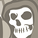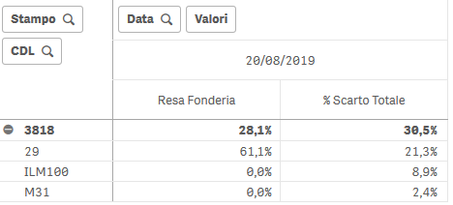# App Development

Announcements
Now Live: Qlik Sense SaaS Simplified Authoring – Analytics Creation for Everyone: READ DETAILS
cancel
Showing results for
Did you mean:Contributor III

## Help with Pivot table values

Hi everyone,

I have a Pivot table with 2 Measures that behave in different way when grouped (I mean the line with the number 3818).As you can see, the left measure (Resa Fonderia) is an Average calculation, while the other one (% Scarto Totale) is a Sum.
I need to have the Sum on both measures.

Below the measure called % Scarto Totale.And here the one called Resa Fonderia.Thanks a lot.

Labels (5)

• ### Pivot Measure

1 Solution

Accepted SolutionsMVP

Try this

``Sum(Aggr(YourExpression, Stampo, CDL, Data))``
6 RepliesMVP

What exactly do you mean when you say you want sum in both measure? Can you provide the numbers you expect to see? Are we only talking about the totals or the individual rows are incorrect as well?Contributor III
Author
Hi,
In the column called Resa Fonderia I'd like to see 61,1% (the Sum of the 3 values you see in the picture) and not 28,1% (the Avg of the 3 values).
Thanks a lot!MVP

Try this

``Sum(Aggr(YourExpression, Stampo, CDL, Data))``Contributor III
Author

I tried but I get 0 in each field.The formula now is:

``Sum(Aggr(Sum([S_ODL.LORDO_FONDERIA])/Sum({<[C_FASI.PRAV]-= {3}, [DT_ARTICOLI.TCICMEDSTD]={">0"}>} [S_ODL.DURATA]/[DT_ARTICOLI.TCICMEDSTD]), Mid([L_ODL.CODPARTE],4,4), [S_ODL.CODCDL], KEY_DATA))``MVP

You will need to create a new field for this in the script

``Mid([L_ODL.CODPARTE],4,4) as ...``

Aggr function's dimension cannot be used with calculated dimensionsContributor III
Author

It works great!

Thank you very much!

(Have you seen the other post I made? Maybe you can help me also there...)